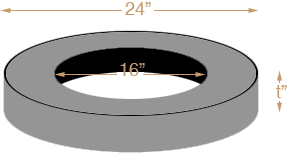SEARCH HOMEMath Central Quandaries & QueriesQuestion from robert, a student: I want to build a volleyball net support. I am using a car tire 24"odx16"id filled with concrete. how much will this weigh? thanksRobert,

You can approximate the concrete shape as a cylinder of diameter 24 inches from which you have removed a concentric cylinder of diameter 16 inches.The volume of a circular cylinder is π r2 t where r is the radius and t is the thickness. To find the volume of concrete first convert all the measurements to feet by dividing each by 12. Find the volume of the large cylinder and subtract the volume of the small cylinder. This will give you a volume in cubic feet. The density of concrete is approximately 150 pounds per cubic foot so multiply the volume by 150 to obtain the approximate weight in pounds.

Good luck in this project,
PennyMath Central is supported by the University of Regina and The Pacific Institute for the Mathematical Sciences.